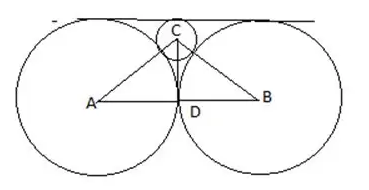### CAT 2019 – Slot 1 – Quantitative Ability – Two circles, each of radius 4 cm, touch externally

\$ 39
99
Monthly
• List Item #1
• List Item #2
Popular

Q. 1: Two circles, each of radius 4 cm, touch externally. Each of these two circles is touched externally by a third circle. If these three circles have a common tangent, then the radius of the third circle, in cm, is
1. π/3
2. 1/√2
3. √2
4. 1

See the figure,In above figure , AD=BD =4 , let radius of 3rd circle = r

So AC = 4+r, CD = 4-r

Using Pythagoras theorem in right angled triangle ADC,

(4+r)^2 = 4^2 + (4-r)^2

16+ r^2 + 8r = 16 + 16 + r^2 – 8r

16r = 16

r =1

##### Q. 2: In an examination, the score of A was 10% less than that of B, the score of B was 25% more than that of C, and the score of C was 20% less than that of D. If A scored 72, then the score of D was

Let the score of B =x

Score of A = x – 10% of x = 0.9x

As per question , score of B = 25% more than C = 5/4 C

And score of C = 20% less than D = 4/5 D

Or D = 5/4 C

So, B = D

Since A = 72, Therefore D = 72/0.9 = 80

Q. 3: A cyclist leaves A at 10 am and reaches B at 11 am. Starting from 10:01 am, every minute a motor cycle leaves A and moves towards B. Forty-five such motor cycles reach B by 11 am. All motor cycles have the same speed. If the cyclist had doubled his speed, how many motor cycles would have reached B by the time the cyclist reached B?
1. 15
2. 23
3. 20
4. 22

Let speed of motor cycles be m and that of cyclist be c.

Since there are 45 motorcycles reaching in 1 hour, so the latest motorcycle must start by 10:15 from A.

Now, cyclist doubles its speed, so it reaches B at 10:30, so maximum motorcycles that can start are from 10:01 to 10:15, so 15 is the correct answer.

Q. 4: The average of 30 integers is 5. Among these 30 integers, there are exactly 20 which do not exceed 5. What is the highest possible value of the average of these 20 integers?
1. 3.5
2. 5
3. 4.5
4. 4

Sum of all 30 integers =5*30 = 150

Minimum possible sum of remaining 10 integers = 10*6 = 60

(as all remaining 10 integers are greater than 5 so their sum will be minimum when they all are equal and each have value =6)

So some of 20 numbers = 150 – 60 = 90

Thus required avg = 90/20 = 4.5

Q. 5:John jogs on track A at 6 kmph and Mary jogs on track B at 7.5 kmph. The total length of tracks A and B is 325 metres. While John makes 9 rounds of track A, Mary makes 5 rounds of track B. In how many seconds will Mary make one round of track A?

Since distance = speed * time, so

Let length of track a be a and that of b be 325-a

9a/6 = 5(325-a)/7.5 (same time)

67.5a = 9750-30a

A = 100 and B = 225 metres

Time taken by mary = 100/7.5*5/18 = 48 sec

Inspiring Education… Assuring Success!!

Ghatkopar | Borivali | Andheri | Online